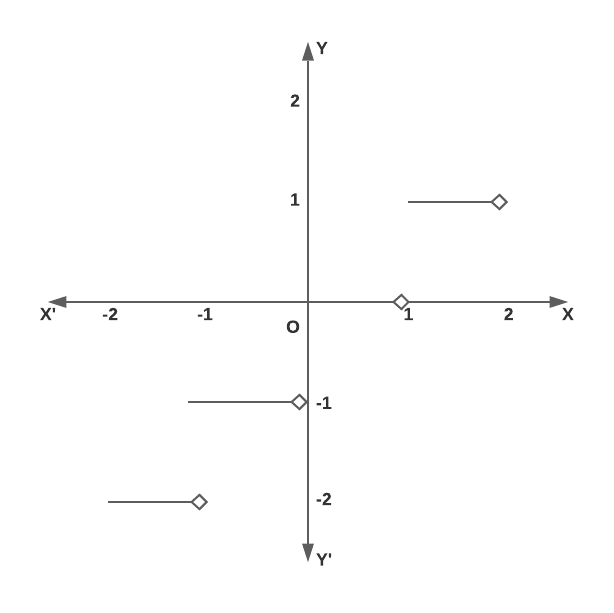# Domain and Range of Greatest Integer Function

Here, you will learn domain and range of greatest integer function and properties of greatest integer function with example.

Let’s begin –

## Greatest Integer Function or Floor Function

For any real number x, we use the symbol [x] or $$\lfloor x \rfloor$$ to denote the greatest integer less than or equal to x. For example,

[2.75] = 2,  =3, [0.74] = 0, [-7.45] = -8 etc.

The Function f : R $$\rightarrow$$ R defined by f(x) = [x] for all x $$\in$$ R is called the greatest integer function or the floor function.

It is also called a step function.

## Domain and Range

Clearly. domain of the greatest integer function is the set of all real numbers and the range is the set Z of all integers as it attains only integer values.

Domain : R

Range : Z

The graph of the greatest integer function is shown in figure.Greatest integer function

## Properties of Greatest Integer Function

If n is an integer and x is a real number between n and n + 1, then

(i) [-n] = -[n]

(ii) [x + k] = [x] + k, for any integer k.

(iii) [-x] = -[x] – 1

(iv) [x] + [-x] = $$\begin{cases} -1, & \text{if}\ x \notin Z \\ 0, & \text{if}\ x \in Z \end{cases}$$

(v) [x] – [-x] = $$\begin{cases} 2[x] + 1, & \text{if}\ x \notin Z \\ 2[x], & \text{if}\ x \in Z \end{cases}$$

(vi) [x] $$\ge$$ k $$\implies$$ x > k, where k $$\in$$ Z

(vii) [x] > k $$\implies$$ x $$\ge$$ k + 1, where k $$\in$$ Z

(viii) [x] > k $$\implies$$ x $$\ge$$ k + 1, where k $$\in$$ Z

(ix) [x] < k $$\implies$$ x < k, where k $$\in$$ Z

(x) [x + y] = [x] + [y + x – [x]] for all x, y $$\in$$ R

Example : If y = 2[x] + 3 & y = 3[x – 2] + 5, then find [x + y] where [.] denotes greatest integer function.

Solution : y = 3[x – 2] + 5 = 3[x] – 1

so 3[x] – 1 = 2[x] + 3

[x] = 4 $$\implies$$ 4 $$\le$$ x < 5

then y = 11

so x + y will lie in the interval [15, 16)

so [x + y] = 15

Hope you learnt domain and range of greatest integer function, learn more concepts of function and practice more questions to get ahead in the competition. Good luck!